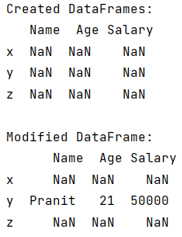# How to fill a DataFrame row by row?

Given a Pandas DataFrame, we have to fill it row by row.
Submitted by Pranit Sharma, on June 18, 2022

Pandas is a special tool which allows us to perform complex manipulations of data effectively and efficiently. Inside pandas, we mostly deal with a dataset in the form of DataFrame. DataFrames are 2-dimensional data structure in pandas. DataFrames consists of rows, columns and the data.

DataFrame may consist of multiple columns and rows where each column may consist of NaN values. Now filling any of the row is a typical task. Pandas allows us to achieve this task by using pandas.DataFrame.loc property and then assigning a Series of elements.

To work with pandas, we need to import pandas package first, below is the syntax:

```import pandas as pd
```

Let us understand with the help of an example,

## Python code to fill a DataFrame row by row

```# Importing pandas package
import pandas as pd

# Creating a DataFrame
df = pd.DataFrame(
columns=['Name','Age','Salary'],
index=['x','y','z']
)

# Display DataFrame with NaN values
print("Created DataFrames:\n",df,"\n")

# Adding data two second row
df.loc['y'] = pd.Series({'Name':'Pranit','Age':21,'Salary':50000})

# Display modified DataFrame
print("Modified DataFrame:\n",df,"\n")
```

Output:Top MCQs